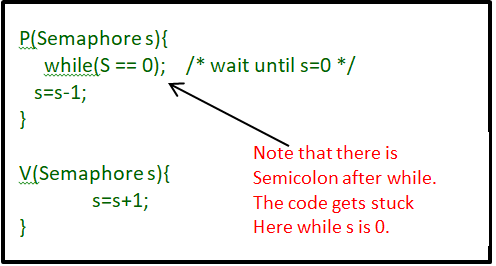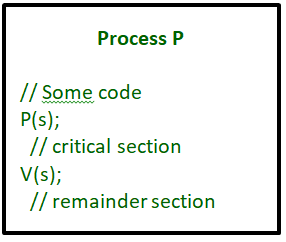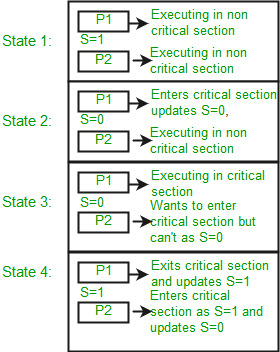Open In App
Related Articles
• Write an Interview Experience
• Operating System Tutorial

# Semaphores in Process Synchronization

Semaphores are just normal variables used to coordinate the activities of multiple processes in a computer system. They are used to enforce mutual exclusion, avoid race conditions and implement synchronization between processes.

The process of using Semaphores provides two operations: wait (P) and signal (V). The wait operation decrements the value of the semaphore, and the signal operation increments the value of the semaphore. When the value of the semaphore is zero, any process that performs a wait operation will be blocked until another process performs a signal operation.

Semaphores are used to implement critical sections, which are regions of code that must be executed by only one process at a time. By using semaphores, processes can coordinate access to shared resources, such as shared memory or I/O devices.

Semaphores are of two types:

1. Binary Semaphore –
This is also known as a mutex lock. It can have only two values – 0 and 1. Its value is initialized to 1. It is used to implement the solution of critical section problems with multiple processes.
2. Counting Semaphore –
Its value can range over an unrestricted domain. It is used to control access to a resource that has multiple instances.

Now let us see how it does so.

First, look at two operations that can be used to access and change the value of the semaphore variable.Some points regarding P and V operation:

1. P operation is also called wait, sleep, or down operation, and V operation is also called signal, wake-up, or up operation.
2. Both operations are atomic and semaphore(s) is always initialized to one. Here atomic means that variable on which read, modify and update happens at the same time/moment with no pre-emption i.e. in-between read, modify and update no other operation is performed that may change the variable.
3. A critical section is surrounded by both operations to implement process synchronization. See the below image. The critical section of Process P is in between P and V operation.Now, let us see how it implements mutual exclusion. Let there be two processes P1 and P2 and a semaphore s is initialized as 1. Now if suppose P1 enters in its critical section then the value of semaphore s becomes 0. Now if P2 wants to enter its critical section then it will wait until s > 0, this can only happen when P1 finishes its critical section and calls V operation on semaphore s.

This way mutual exclusion is achieved. Look at the below image for details which is a Binary semaphore.Implementation: Binary semaphores

## C++

 `struct` `semaphore {``  ` `    ``enum` `value(0, 1);` `    ``// q contains all Process Control Blocks (PCBs)``    ``// corresponding to processes got blocked``    ``// while performing down operation.``    ``Queue q;` `};``P(semaphore s)``{``    ``if` `(s.value == 1) {``        ``s.value = 0;``    ``}``    ``else` `{``        ``// add the process to the waiting queue``        ``q.push(P) sleep();``    ``}``}``V(Semaphore s)``{``    ``if` `(s.q is empty) {``        ``s.value = 1;``    ``}``    ``else` `{` `        ``// select a process from waiting queue``        ``Process p = q.front();``        ``// remove the process from waiting as it has been``        ``// sent for CS``        ``q.pop();``        ``wakeup(p);``    ``}``}`

## Java

 `import` `java.util.*;` `class` `Semaphore {``    ``public` `enum` `Value { Zero, One }``    ``public` `Queue q = ``new` `LinkedList();``    ``public` `Value value = Value.One;` `    ``public` `void` `P(Semaphore s, Process p)``    ``{``        ``if` `(s.value == Value.One) {``            ``s.value = Value.Zero;``        ``}``        ``else` `{``            ``// add the process to the waiting queue``            ``q.add(p);``            ``p.Sleep();``        ``}``    ``}` `    ``public` `void` `V(Semaphore s)``    ``{``        ``if` `(s.q.size() == ``0``) {``            ``s.value = Value.One;``        ``}``        ``else` `{``            ``// select a process from waiting queue``            ``Process p = q.peek();``            ``// remove the process from waiting as it has``            ``// been sent for CS``            ``q.remove();``            ``p.Wakeup();``        ``}``    ``}``}`

## Python3

 `from` `enum ``import` `Enum``from` `queue ``import` `Queue` `class` `Semaphore:``    ``class` `Value(Enum):``        ``Zero ``=` `0``        ``One ``=` `1` `    ``def` `__init__(``self``):``        ``self``.q ``=` `Queue()``        ``self``.value ``=` `Semaphore.Value.One` `    ``def` `P(``self``, s, p):``        ``if` `s.value ``=``=` `Semaphore.Value.One:``            ``s.value ``=` `Semaphore.Value.Zero``        ``else``:``            ``# add the process to the waiting queue``            ``s.q.put(p)``            ``p.Sleep()` `    ``def` `V(``self``, s):``        ``if` `s.q.qsize() ``=``=` `0``:``            ``s.value ``=` `Semaphore.Value.One``        ``else``:``            ``# select a process from waiting queue``            ``p ``=` `s.q.queue[``0``]``            ``# remove the process from waiting as it has``            ``# been sent for CS``            ``s.q.get()``            ``p.Wakeup()`

## C#

 `using` `System.Collections.Generic;` `class` `Semaphore {``    ``public` `enum` `value { Zero, One }``    ``public` `Queue q = ``new` `Queue();` `    ``public` `void` `P(Semaphore s, Process p) {``        ``if` `(s.value == value.One) {``            ``s.value = value.Zero;``        ``} ``else` `{``            ``// add the process to the waiting queue``            ``q.Enqueue(p);``            ``p.Sleep();``        ``}``    ``}` `    ``public` `void` `V(Semaphore s) {``        ``if` `(s.q.Count == 0) {``            ``s.value = value.One;``        ``} ``else` `{``            ``// select a process from waiting queue``            ``Process p = q.Peek();``            ``// remove the process from waiting as it has been``            ``// sent for CS``            ``q.Dequeue();``            ``p.Wakeup();``        ``}``    ``}``}`

## Javascript

 `class Semaphore {``  ``constructor() {``    ``this``.value = 0;``    ``// q contains all Process Control Blocks (PCBs)``    ``// corresponding to processes got blocked``    ``// while performing down operation.``    ``this``.q = [];``  ``}` `  ``P() {``    ``if` `(``this``.value == 1) {``      ``this``.value = 0;``    ``} ``else` `{``      ``// add the process to the waiting queue``      ``this``.q.push(P);``      ``sleep();``    ``}``  ``}` `  ``V() {``    ``if` `(``this``.q.length == 0) {``      ``this``.value = 1;``    ``} ``else` `{``      ``// select a process from waiting queue``      ``let p = ``this``.q.shift();``      ``// remove the process from waiting as it has been``      ``// sent for CS``      ``wakeup(p);``    ``}``  ``}``}`

## C

 `#include ``#include``#include``struct` `semaphore{``    ``Queue q;``    ``int` `value;``};` `void` `P(``struct` `semaphore s)``{``    ``if` `(s.value == 1) {``        ``s.value = 0;``    ``}``    ``else` `{``        ``s.q.push(P);``        ``sleep();``    ``}``}` `void` `V(Semaphore s)``{``    ``if` `(s.q is empty) {``        ``s.value = 1;``    ``}``    ``else` `{`` ` `        ``// Get a process from the Waiting Queue``        ``Process p = q.front();``        ``// Remove the process from waiting``        ``q.pop();``        ``wakeup(p);``    ``}``}``int` `main() {``    ``printf``(``"This is Hemish!!"``);``  ` `    ``// This code is Contributed by Himesh Singh Chauhan``    ``return` `0;``}`

The description above is for binary semaphore which can take only two values 0 and 1 and ensure mutual exclusion. There is one other type of semaphore called counting semaphore which can take values greater than one.

Now suppose there is a resource whose number of instances is 4. Now we initialize S = 4 and the rest is the same as for binary semaphore. Whenever the process wants that resource it calls P or waits for function and when it is done it calls V or signal function. If the value of S becomes zero then a process has to wait until S becomes positive. For example, Suppose there are 4 processes P1, P2, P3, P4, and they all call wait operation on S(initialized with 4). If another process P5 wants the resource then it should wait until one of the four processes calls the signal function and the value of semaphore becomes positive.

Limitations :

1. One of the biggest limitations of semaphore is priority inversion.
2. Deadlock, suppose a process is trying to wake up another process that is not in a sleep state. Therefore, a deadlock may block indefinitely.
3. The operating system has to keep track of all calls to wait and signal the semaphore.

Problem in this implementation of a semaphore:

The main problem with semaphores is that they require busy waiting, If a process is in the critical section, then other processes trying to enter the critical section will be waiting until the critical section is not occupied by any process. Whenever any process waits then it continuously checks for semaphore value (look at this line while (s==0); in P operation) and waste CPU cycle.

There is also a chance of “spinlock” as the processes keep on spins while waiting for the lock. In order to avoid this another implementation is provided below.

Implementation: Counting semaphore

## CPP

 `struct` `Semaphore {` `    ``int` `value;` `    ``// q contains all Process Control Blocks(PCBs)``    ``// corresponding to processes got blocked``    ``// while performing down operation.``    ``Queue q;` `};``P(Semaphore s)``{``    ``s.value = s.value - 1;``    ``if` `(s.value < 0) {` `        ``// add process to queue``        ``// here p is a process which is currently executing``        ``q.push(p);``        ``block();``    ``}``    ``else``        ``return``;``}` `V(Semaphore s)``{``    ``s.value = s.value + 1;``    ``if` `(s.value <= 0) {` `        ``// remove process p from queue``        ``Process p = q.pop();``        ``wakeup(p);``    ``}``    ``else``        ``return``;``}`

## Java

 `import` `java.util.LinkedList;``import` `java.util.Queue;` `// semaphore class``class` `Semaphore {` `  ``// our value``  ``int` `value;``    ``Queue q;` `    ``public` `Semaphore(``int` `value)``    ``{``        ``this``.value = value;``        ``q = ``new` `LinkedList<>();``    ``}` `    ``public` `void` `P(Process p)``    ``{``        ``value--;``        ``if` `(value < ``0``) {``            ``q.add(p);``            ``p.block();``        ``}``    ``}` `    ``public` `void` `V()``    ``{``        ``value++;``        ``if` `(value <= ``0``) {``            ``Process p = q.remove();``            ``p.wakeup();``        ``}``    ``}``}`

## C#

 `using` `System.Collections.Generic;` `public` `class` `Semaphore {``    ``public` `int` `value;``    ``// q contains all Process Control Blocks(PCBs)``    ``// corresponding to processes got blocked``    ``// while performing down operation.``    ``Queue q = ``new` `Queue();` `    ``public` `void` `P(Process p)``    ``{``        ``value--;``        ``if` `(value < 0) {``            ``// add process to queue``            ``q.Enqueue(p);``            ``p.block();``        ``}``    ``}` `    ``public` `void` `V()``    ``{``        ``value++;``        ``if` `(value <= 0) {``            ``// remove process p from queue``            ``Process p = q.Dequeue();``            ``p.wakeup();``        ``}``    ``}``}`

## Python3

 `import` `heapq``# Global Variable to track the Processes going into Critical Section``COUNTER``=``1` `class` `Semaphore:``    ``def` `__init__(``self``,value):``        ``# Value of the Semaphore passed to the Constructor``        ``self``.value``=``value``        ``# The Waiting queue which will be using the heapq module of Python``        ``self``.q``=``list``()` `    ``def` `getSemaphore(``self``):``        ``''' Function to print the Value of the Semaphore Variable '''``        ``print``(f``"Semaphore Value: {self.value}"``)``        ` `def` `block(process):``    ``print``(f``"Process {process} Blocked."``)``    ` `def` `wakeup(process):``    ``print``(f``"Process {process} Waked Up and Completed it's work."``)` `def` `P(s):``    ``global` `COUNTER``    ``s.value``=``s.value``-``1``    ``if``(s.value<``0``):``        ``heapq.heappush(s.q,COUNTER)``        ``block(COUNTER)``    ``else``:``        ``print``(f``'Process {COUNTER} gone inside the Critical Section.'``)``        ``COUNTER``+``=``1``        ``return``    ` `def` `V(s):``    ``global` `COUNTER``    ``s.value``=``s.value``+``1``    ``if``(s.value<``=``0``):``        ``p``=``heapq.heappop(s.q)``        ``wakeup(p)``        ``COUNTER``-``=``1``    ``else``:``        ``print``(f``"Process {COUNTER} completed it's work."``)``        ``COUNTER``-``=``1``        ``return``    ` `# Can Pass the Value of the Counting Semaphore to the Class Constructor` `# Example for Counting Semaphore value as 2``s1``=``Semaphore(``2``)``s1.getSemaphore()` `P(s1)``s1.getSemaphore()` `P(s1)``s1.getSemaphore()` `P(s1)``s1.getSemaphore()` `V(s1)``s1.getSemaphore()` `V(s1)``s1.getSemaphore()` `V(s1)``s1.getSemaphore()` `# This Code is Contributed by Himesh Singh Chauhan`

In this implementation whenever the process waits it is added to a waiting queue of processes associated with that semaphore. This is done through the system call block() on that process. When a process is completed it calls the signal function and one process in the queue is resumed. It uses the wakeup() system call.

• A simple and effective mechanism for process synchronization
• Supports coordination between multiple processes
• Provides a flexible and robust way to manage shared resources.
• It can be used to implement critical sections in a program.
• It can be used to avoid race conditions.